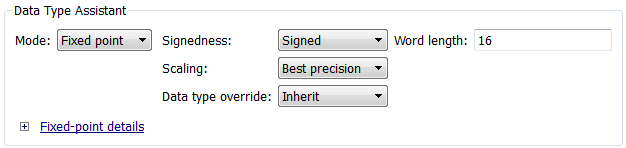## Maximize Precision

Precision is limited by slope. To achieve maximum precision, you should make the slope as small as possible while keeping the range adequately large. The bias is adjusted in coordination with the slope.

Assume the maximum and minimum real-world values are given by max(V) and min(V), respectively. These limits might be known based on physical principles or engineering considerations. To maximize the precision, you must decide upon a rounding scheme and whether overflows saturate or wrap. To simplify matters, this example assumes the minimum real-world value corresponds to the minimum encoded value, and the maximum real-world value corresponds to the maximum encoded value. Using the encoding scheme described in Scaling, these values are given by

`$\begin{array}{c}\mathrm{max}\left(V\right)=F{2}^{E}\left(\mathrm{max}\left(Q\right)\right)+B\\ \mathrm{min}\left(V\right)=F{2}^{E}\left(\mathrm{min}\left(Q\right)\right)+B.\end{array}$`

Solving for the slope, you get

`$F{2}^{E}=\frac{\mathrm{max}\left(V\right)-\mathrm{min}\left(V\right)}{\mathrm{max}\left(Q\right)-\mathrm{min}\left(Q\right)}=\frac{\mathrm{max}\left(V\right)-\mathrm{min}\left(V\right)}{{2}^{ws}-1}.$`

This formula is independent of rounding and overflow issues, and depends only on the word size, ws.

Padding with trailing zeros involves extending the least significant bit (LSB) of a number with extra bits. This method involves going from low precision to higher precision.

For example, suppose two numbers are subtracted from each other. First, the exponents must be aligned, which typically involves a right shift of the number with the smaller value. In performing this shift, significant digits can “fall off” to the right. However, when the appropriate number of extra bits is appended, the precision of the result is maximized. Consider two 8-bit fixed-point numbers that are close in value and subtracted from each other:

`$1.0000000×{2}^{q}-1.1111111×{2}^{q-1},$`

where q is an integer. To perform this operation, the exponents must be equal:

`$\begin{array}{c}1.0000000×{2}^{q}\\ \frac{-0.1111111×{2}^{q}}{\text{\hspace{0.17em}}\text{\hspace{0.17em}}0.0000001×{2}^{q}}.\end{array}$`

If the top number is padded by two zeros and the bottom number is padded with one zero, then the above equation becomes

`$\begin{array}{c}1.000000000×{2}^{q}\\ \frac{-0.111111110×{2}^{q}}{\text{\hspace{0.17em}}\text{\hspace{0.17em}}0.000000010×{2}^{q}},\end{array}$`

which produces a more precise result. An example of padding with trailing zeros in a Simulink® model is illustrated in Digital Controller Realization.

### Constant Scaling for Best Precision

The following fixed-point Simulink blocks provide a mode for scaling parameters whose values are constant vectors or matrices:

This scaling mode is based on binary-point-only scaling. Using this mode, you can scale a constant vector or matrix such that a common binary point is found based on the best precision for the largest value in the vector or matrix.

Constant scaling for best precision is available only for fixed-point data types with unspecified scaling. All other fixed-point data types use their specified scaling. You can use the Data Type Assistant (see Specify Data Types Using Data Type Assistant) on a block dialog box to enable the best precision scaling mode.

1. On a block dialog box, click the button.

The Data Type Assistant appears.

2. In the Data Type Assistant, and from the Mode list, select ```Fixed point```.

The Data Type Assistant displays additional options associated with fixed-point data types.

3. From the Scaling list, select ```Best precision```.To understand how you might use this scaling mode, consider a 3-by-3 matrix of doubles, M, defined as

``` 3.3333e-003 3.3333e-004 3.3333e-005 3.3333e-002 3.3333e-003 3.3333e-004 3.3333e-001 3.3333e-002 3.3333e-003```

Now suppose you specify M as the value of the Gain parameter for a Gain block. The results of specifying your own scaling versus using the constant scaling mode are described here:

• Specified Scaling

Suppose the matrix elements are converted to a signed, 10-bit generalized fixed-point data type with binary-point-only scaling of `2`-7 (that is, the binary point is located seven places to the left of the right most bit). With this data format, M becomes

```0 0 0 3.1250e-002 0 0 3.3594e-001 3.1250e-002 0```

Note that many of the matrix elements are zero, and for the nonzero entries, the scaled values differ from the original values. This is because a double is converted to a binary word of fixed size and limited precision for each element. The larger and more precise the conversion data type, the more closely the scaled values match the original values.

• Constant Scaling for Best Precision

If M is scaled based on its largest matrix value, you obtain

```2.9297e-003 0 0 3.3203e-002 2.9297e-003 0 3.3301e-001 3.3203e-002 2.9297e-003```

Best precision would automatically select the fraction length that minimizes the quantization error. Even though precision was maximized for the given word length, quantization errors can still occur. In this example, a few elements still quantize to zero.

## SupportGet trial now